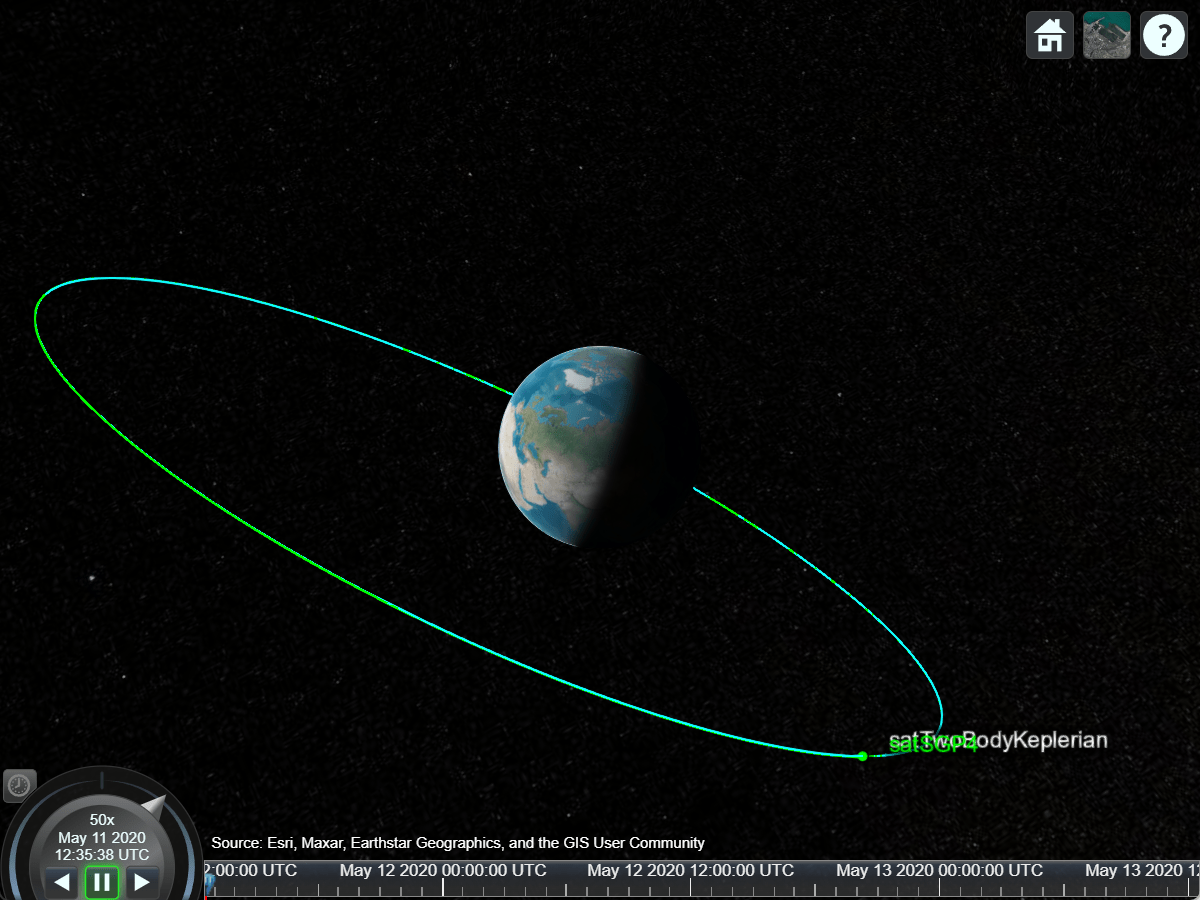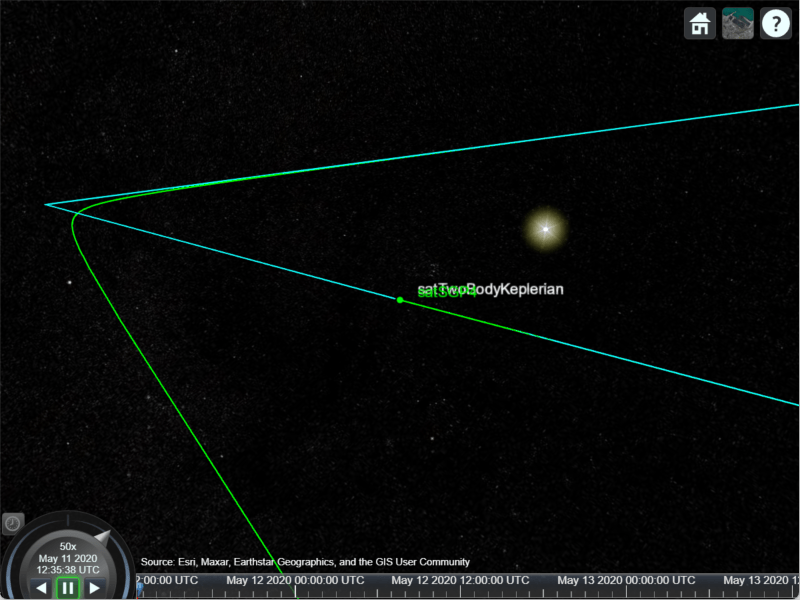# Comparison of Orbit Propagators

This example compares the orbits predicted by the Two-Body-Keplerian, Simplified General Perturbations-4 (SGP4) and Simplified Deep-Space Perturbations-4 (SDP4) orbit propagators. An orbit propagator is a solver that calculates the position and velocity of an object whose motion is predominantly influenced by gravity from celestial bodies. The Two-Body-Keplerian orbit propagator is based on the relative two-body model that assumes a spherical gravity field for the Earth and neglects third body effects and other environmental perturbations, and hence, is the least accurate. The SGP4 orbit propagator accounts for secular and periodic orbital perturbations caused by Earth's geometry and atmospheric drag, and is applicable to near-Earth satellites whose orbital period is less than 225 minutes. The SDP4 orbit propagator builds upon SGP4 by accounting for solar and lunar gravity, and is applicable to satellites whose orbital period is greater than or equal to 225 minutes. The default orbit propagator for `satelliteScenario` is SGP4 for satellites whose orbital period is less than 225 minutes, and SDP4 otherwise.

### Create a Satellite Scenario

Create a satellite scenario by using the `satelliteScenario` function. Set the start time to 11-May-2020 12:35:38 PM UTC, and the stop time to 13-May-2020 12:35:38 PM UTC, by using the `datetime` function. Set the sample time to 60 seconds.

```startTime = datetime(2020,5,11,12,35,38); stopTime = startTime + days(2); sampleTime = 60; sc = satelliteScenario(startTime,stopTime,sampleTime)```
```sc = satelliteScenario with properties: StartTime: 11-May-2020 12:35:38 StopTime: 13-May-2020 12:35:38 SampleTime: 60 Viewers: [0×0 matlabshared.satellitescenario.Viewer] Satellites: [] GroundStations: [] AutoShow: 1 ```

### Add Satellites to the Satellite Scenario

Add three satellites to the satellite scenario from the two-line element (TLE) file `eccentricOrbitSatellite.tle` by using the `satellite` function. TLE is a data format used for encoding the orbital elements of an Earth-orbiting object defined at a specific time. Assign a Two-Body-Keplerian orbit propagator to the first satellite, SGP4 to the second satellite, and SDP4 to the third satellite.

```tleFile = "eccentricOrbitSatellite.tle"; satTwoBodyKeplerian = satellite(sc,tleFile, ... "Name","satTwoBodyKeplerian", ... "OrbitPropagator","two-body-keplerian")```
```satTwoBodyKeplerian = Satellite with properties: Name: "satTwoBodyKeplerian" ID: 1 ConicalSensors: [] Gimbals: [] Transmitters: [] Receivers: [] Accesses: [] GroundTrack: [1×1 matlabshared.satellitescenario.GroundTrack] Orbit: [1×1 matlabshared.satellitescenario.Orbit] OrbitPropagator: "two-body-keplerian" MarkerColor: [1 0 0] MarkerSize: 10 ShowLabel: 1 LabelFontColor: [1 0 0] LabelFontSize: 15 ```
```satSGP4 = satellite(sc,tleFile, ... "Name","satSGP4", ... "OrbitPropagator","sgp4")```
```satSGP4 = Satellite with properties: Name: "satSGP4" ID: 2 ConicalSensors: [] Gimbals: [] Transmitters: [] Receivers: [] Accesses: [] GroundTrack: [1×1 matlabshared.satellitescenario.GroundTrack] Orbit: [1×1 matlabshared.satellitescenario.Orbit] OrbitPropagator: "sgp4" MarkerColor: [1 0 0] MarkerSize: 10 ShowLabel: 1 LabelFontColor: [1 0 0] LabelFontSize: 15 ```
```satSDP4 = satellite(sc,tleFile, ... "Name","satSDP4", ... "OrbitPropagator","sdp4")```
```satSDP4 = Satellite with properties: Name: "satSDP4" ID: 3 ConicalSensors: [] Gimbals: [] Transmitters: [] Receivers: [] Accesses: [] GroundTrack: [1×1 matlabshared.satellitescenario.GroundTrack] Orbit: [1×1 matlabshared.satellitescenario.Orbit] OrbitPropagator: "sdp4" MarkerColor: [1 0 0] MarkerSize: 10 ShowLabel: 1 LabelFontColor: [1 0 0] LabelFontSize: 15 ```

### Visualize the Satellites and their Orbits

Launch a satellite scenario viewer and visualize the satellite scenario by using the `satelliteScenarioViewer` function. Set the visualizations of `satTwoBodyKeplerian` to red, `satSGP4` to green, and `satSDP4` to magenta.

```v = satelliteScenarioViewer(sc); satSGP4.MarkerColor = [0 1 0]; satSGP4.Orbit.LineColor = [0 1 0]; satSGP4.LabelFontColor = [0 1 0]; satSDP4.MarkerColor = [1 0 1]; satSDP4.Orbit.LineColor = [1 0 1]; satSDP4.LabelFontColor = [1 0 1];```Focus the camera on `satTwoBodyKeplerian` by using the `camtarget` function.

`camtarget(v,satTwoBodyKeplerian);`

Left-click anywhere inside the satellite scenario viewer window and move the mouse while holding the click to pan the camera. Adjust the zoom level using the scroll wheel to bring all three satellites into view.### Visualize a Dynamic Animation of the Satellite Movement

Visualize the movement of the satelliles by using the `play` function on the satellite scenario. The `play` function simulates the satellite scenario from the specified `StartTime` to `StopTime` using a step size specified by `SampleTime`, and plays the results on the satellite scenario viewer.

`play(sc)`

Use the playback controls located at the bottom of the satellite scenario viewer window to control the playback speed and direction. Focus the camera again on `satTwoBodyKeplerian` by using the `camtarget` function, and bring all three satellites into view by adjusting the zoom level.

`camtarget(v,satTwoBodyKeplerian);`

The positions of the three satellites diverge over time.### Obtain the Position and Velocity History of the Satellites

Return the position and velocity history of the satellites in the Geocentric Celestial Reference Frame (GCRF) by using the `states` function.

```[positionTwoBodyKeplerian,velocityTwoBodyKeplerian,time] = states(satTwoBodyKeplerian); [positionSGP4,velocitySGP4] = states(satSGP4); [positionSDP4,velocitySDP4] = states(satSDP4);```

### Plot Magnitude of Relative Position with Respect to Two-Body-Keplerian Prediction

Calculate the magnitude of the relative position of `satSGP4` and `satSDP4` with respect to `satTwoBodyKeplerian` by using the `vecnorm` function.

```sgp4RelativePosition = vecnorm(positionSGP4 - positionTwoBodyKeplerian,2,1); sdp4RelativePosition = vecnorm(positionSDP4 - positionTwoBodyKeplerian,2,1);```

Plot the magnitude of the relative positions in kilometers of `satSGP4` and `satSDP4` with respect to that of `satTwoBodyKeplerian` by using the `plot` function.

```sgp4RelativePositionKm = sgp4RelativePosition/1000; sdp4RelativePositionKm = sdp4RelativePosition/1000; plot(time,sgp4RelativePositionKm,time,sdp4RelativePositionKm) xlabel("Time") ylabel("Relative position (km)") legend("SGP4","SDP4")```The initial relative position of `satSGP4` is non-zero and that of `satSDP4` is zero because the initial positions of `satTwoBodyKeplerian` and `satSDP4` are calculated from the TLE file using the SDP4 orbit propagator, while the initial position of `satSGP4` is calculated using the SGP4 orbit propagator. Over time, the position of `satSDP4` deviates from that of `satTwoBodyKeplerian` because the subsequent positions of the former are calcluated using the SDP4 orbit propagator, while those of the latter are calculated using the Two-Body-Keplerian orbit propagator. The SDP4 orbit propagator provides higher precision because unlike the Two-Body-Keplerian orbit propagator, it accounts for oblateness of the Earth, atmospheric drag, and gravity from the sun and the moon.

### Plot Magnitude of Relative Velocity with Respect to Two-Body-Keplerian Prediction

Calculate the magnitude of the relative velocity of `satSGP4` and `satSDP4` with respect to `satTwoBodyKeplerian` by using the `vecnorm` function.

```sgp4RelativeVelocity = vecnorm(velocitySGP4 - velocityTwoBodyKeplerian,2,1); sdp4RelativeVelocity = vecnorm(velocitySDP4 - velocityTwoBodyKeplerian,2,1);```

Plot the magnitude of the relative velocities in meters per second of `satSGP4` and `satSDP4` with respect to `satTwoBodyKeplerian` by using the `plot` function.

```plot(time,sgp4RelativeVelocity,time,sdp4RelativeVelocity) xlabel("Time") ylabel("Velocity deviation (m/s)") legend("SGP4","SDP4")```The initial relative velocity of `satSDP4` is zero because similar to the initial position, the initial velocity of `satTwoBodyKeplerian` and `satSDP4` are also calculated from the TLE file using the SDP4 orbit propagator. Over time, the velocity of `satSDP4` deviates from that of `satTwoBodyKeplerian` because at all other times, the velocity of `satTwoBodyKeplerian` is calculated using the Two-Body-Keplerian orbit propagator, which has lower precision when compared to that of the SDP4 orbit propagator that is used for calculating the velocity of `satSDP4`. The spikes correspond to the periapsis (the closest point in the orbit from the center of mass of the Earth), where the magnitudes of the velocity errors are pronounced.

### Conclusion

The deviations in the plots are the result of varying levels of accuracy of the three orbit propagators. The Two-Body-Keplerian orbit propagator is the least accurate as it assumes that the gravity field of the Earth is spherical, and also neglects all other sources of orbital perturbations. The SGP4 orbit propagator is more accurate as it accounts for the oblateness of the Earth and atmospheric drag. The SDP4 orbit propagator is the most accurate among the three because it also accounts for solar and lunar gravity, which is more pronounced in this example because the orbital period is greater than 225 minutes, thereby taking the satellite farther away from the Earth.

## Related Examples